# Category: Tutorials

## Matplotlib Histogram

Matplotlib can be used to create histograms. A histogram shows the frequency on the vertical axis and the horizontal axis is another dimension. Usually it has bins, where every bin has a minimum and maximum value. Each bin also has a frequency between x and infinite.

Related course

Matplotlib histogram example
Below we show the most minimal Matplotlib histogram:

Output: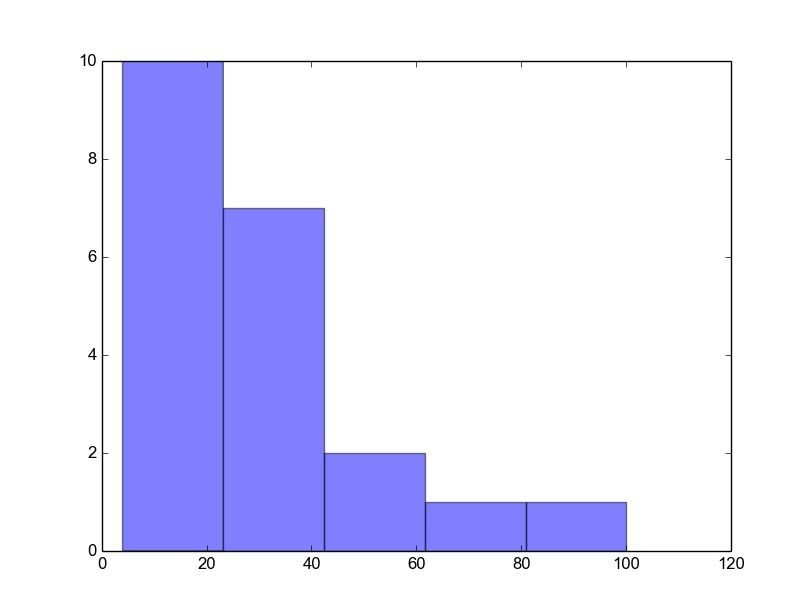Python histogram

A complete matplotlib python histogram
Many things can be added to a histogram such as a fit line, labels and so on. The code below creates a more advanced histogram.

Output: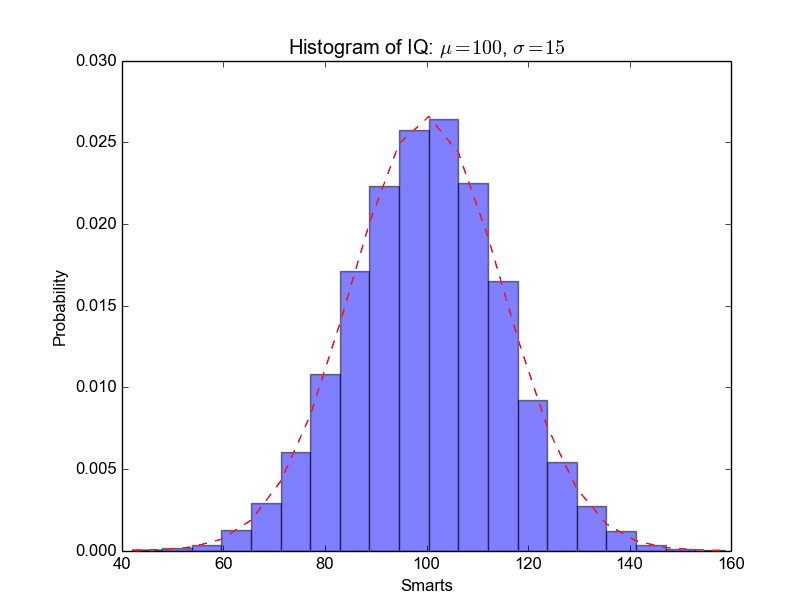python_histogram

## Matplotlib Bar chart

Matplotlib may be used to create bar charts. You might like the Matplotlib gallery.

Matplotlib is a python library for visualizing data. You can use it to create bar charts in python. Installation of matplot is on pypi, so just use pip: pip install matplotlib

The course below is all about data visualization:

Related course:
Data Visualization with Matplotlib and Python

## Bar chart code

A bar chart shows values as vertical bars, where the position of each bar indicates the value it represents. matplot aims to make it as easy as possible to turn data into Bar Charts.

A bar chart in matplotlib made from python code. The code below creates a bar chart:

Output: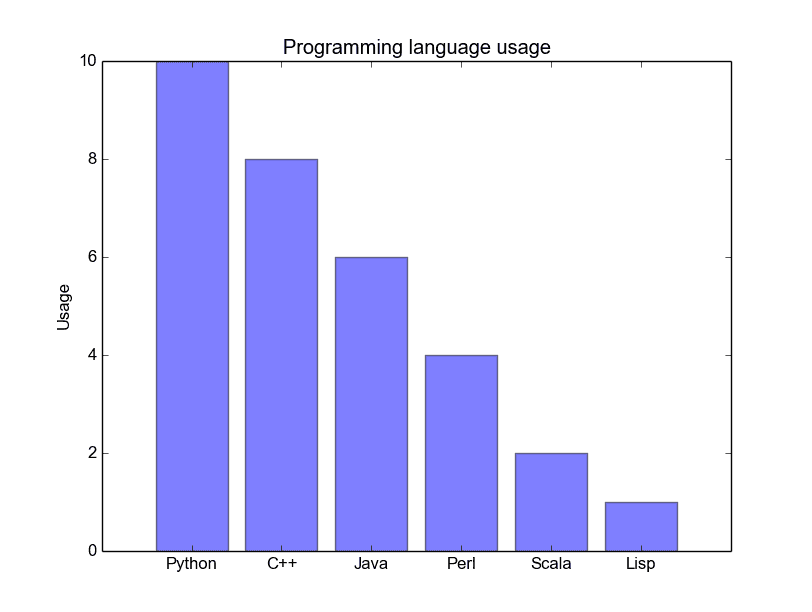Python Bar Chart

Matplotlib charts can be horizontal, to create a horizontal bar chart:

Output: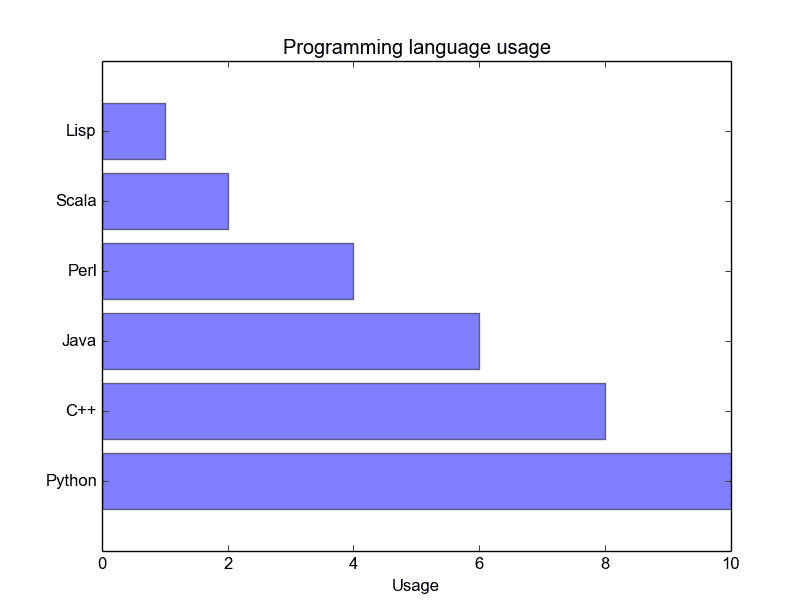Bar chart horizontal

## Bar chart comparison

You can compare two data series using this Matplotlib code:

Output: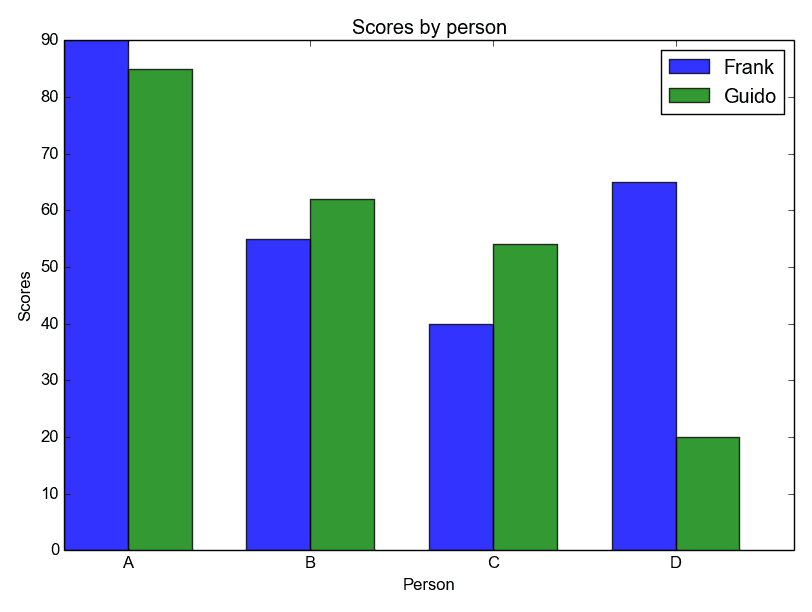Python Bar Chart comparison

## Stacked bar chart

The example below creates a stacked bar chart with Matplotlib. Stacked bar plots show diffrent groups together.

Output:## Matplotlib Pie chart

Matplotlib supports pie charts using the pie() function. You might like the Matplotlib gallery.

The matplotlib module can be used to create all kinds of plots and charts with Python. A pie chart is one of the charts it can create, but it is one of the many.

Related course: Data Visualization with Matplotlib and Python

## Matplotlib pie chart

First import plt from the matplotlib module with the line import matplotlib.pyplot as plt
Then you can use the method plt.pie() to create a plot.

The code below creates a pie chart:

The above code has the following output: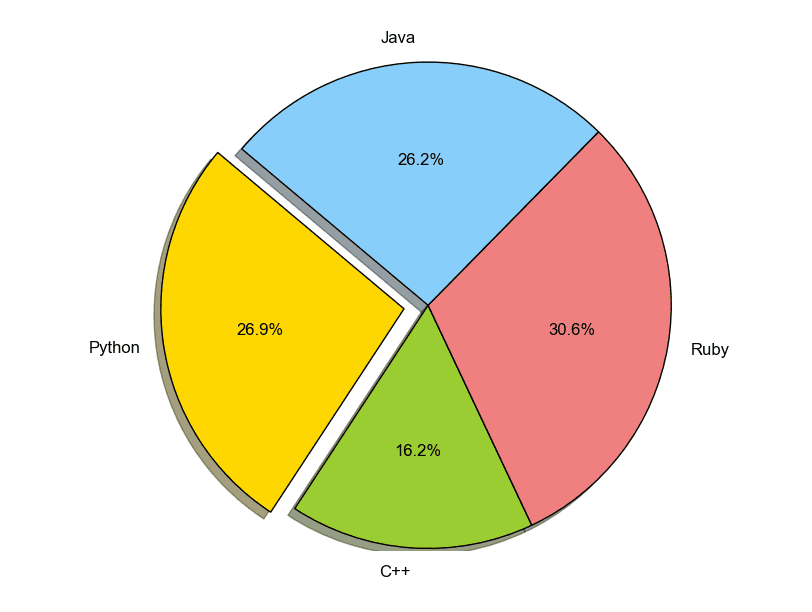pie chart python

You can define it’s sizes, which parts should explode (distance from center), which labels it should have and which colors it should have.

## Matplotlib pie chart legend

To add a legend use the plt.legend() function. This adds a legend on top of the plot.

It outputs this plot: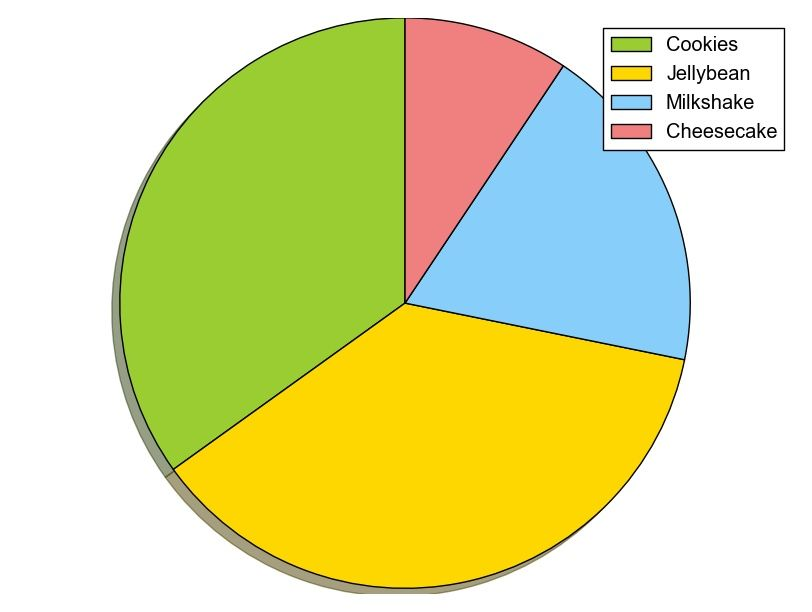python pie chart

While making the plot, don’t forget to call the method .show().